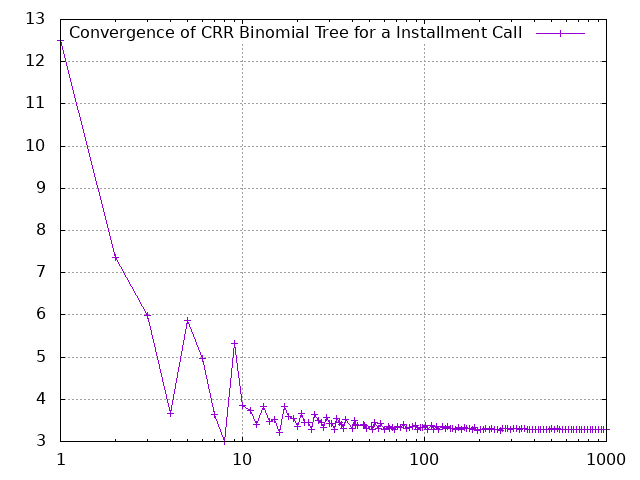# Option Pricing Calculator based on Cox, Ross and Rubinstein

For details on option pricing using trees see Cox, John C., Stephen A. Ross, and Mark Rubinstein. "Option pricing: A simplified approach." Journal of financial Economics 7.3 (1979): 229-263. For introduction to different type of options see

Parameters
 Time to maturity Floating point value, e.g. 1.25 for 15 month Timesteps Integer value, e.g. 4 Volatility Floating point value, e.g. 0.25 for 25% volatility Risk-free interest Floating point value, e.g. 0.5 for 5.0% interest rate Asset price at time zero Strike price Dividend yield Floating point value, e.g. 0.03 for 3.0% dividend yield. This an non-continous dividend with ex-dividend dates on each tree step! Option type European Call European Put American Call American Put Installment Call Installment Put Installment parameters Number of installments Installment premium Fixed premium Proportional premium Absolut Euro premiumPercentage of underlying account Deducted from AV Premium is taken from underlying account (currently only for prop. prem.) Rational behaviour If option value < premium, holder exits General Parametrs Default Calculation Convergence Regression Test Default binomial tree calculation Simulation of convergence with increasing tree steps Regression Test Runs several predefined valuations

## Convergence of CRR Binomial Tree for a Installment Call

Option price = 3.282481 (1000 timesteps / time 0.082607 secs)crr (at) neurolab (dot) de